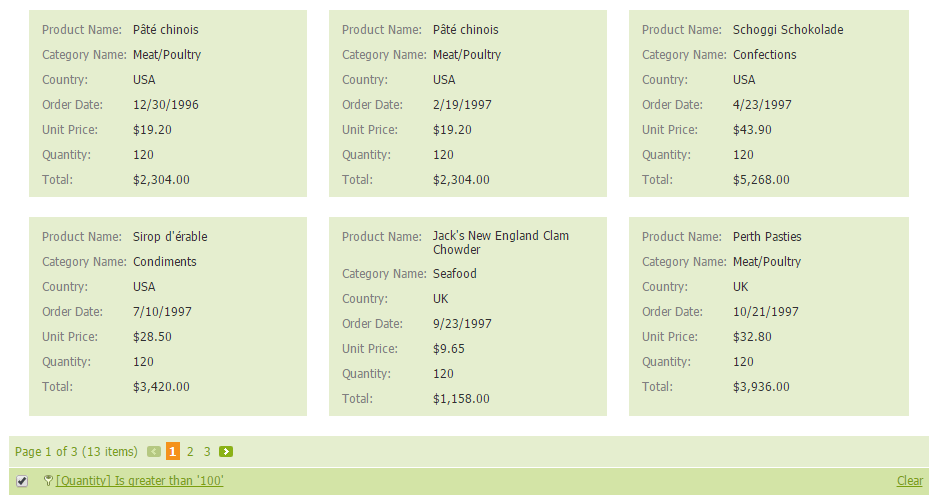Assume that ASPxCardView is bound to a data table that contains the “UnitPrice” and “Quantity” fields. Since there is no field in the data source that represents the total sum, you can calculate it manually as follows: UnitPrice*Quantity. The following example adds an unbound column to the ASPxCardView control that displays each order’s total sum.

The image below shows the result.``````    protected void CardView_Init(object sender, EventArgs e) {
CardViewTextColumn colTotal = new CardViewTextColumn();
colTotal.Caption = "Total";
colTotal.FieldName = "Total";
colTotal.UnboundType = DevExpress.Data.UnboundColumnType.Integer;
colTotal.VisibleIndex = CardView.Columns.Count;
colTotal.PropertiesTextEdit.DisplayFormatString = "c2";